Author: Oscar Cronquist Article last updated on October 26, 2022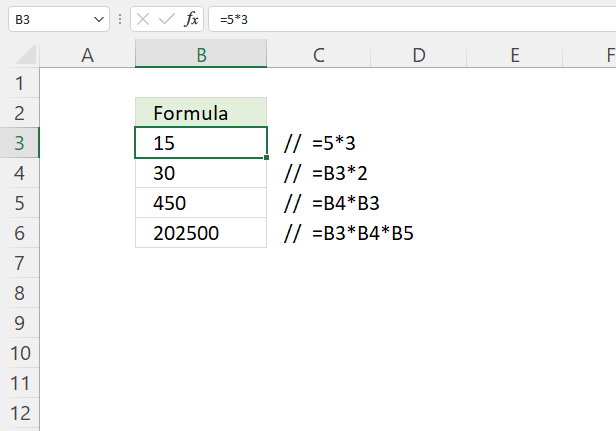The asterisk character allows you to multiply numbers and boolean values in an Excel formula. It can also be used as a wildcard character in quite a few Excel functions.

I will in this article demonstrate when you can use the asterisk character.

## 1. How to multiply numbers in an Excel formulaYou can use numbers in an Excel formula, they are named constants or hard-coded numbers since they don't change unless the Excel user changes them.

To multiply constants simply use the asterisk character to calculate the multiplication between two constants. Here is a simple example:

Formula in cell B3:

=5*3

The formula is calculated as soon as you press Enter on the keyboard, and the result is shown in the cell. The result from multiplication is called a product.

Use key F9 to calculate the result of any calculation, not just multiplication, here is how:

1. Select the part in the formula you want to calculate using the mouse or keyboard.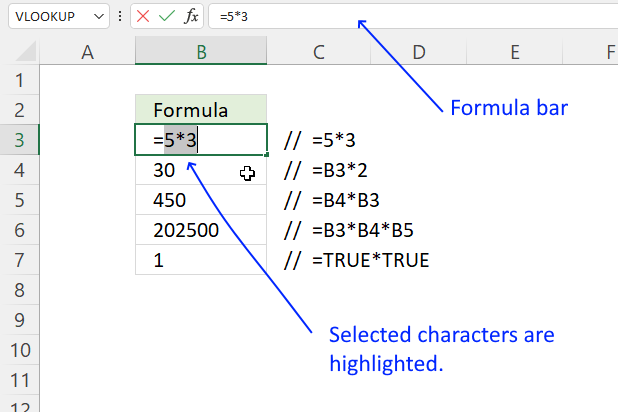2. Press function key F9.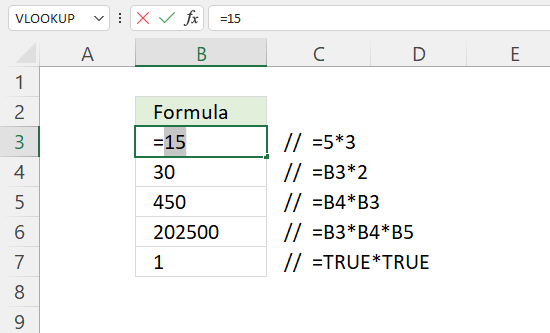The cell and the formula bar show the result.

## 2. How to multiply a number and cell value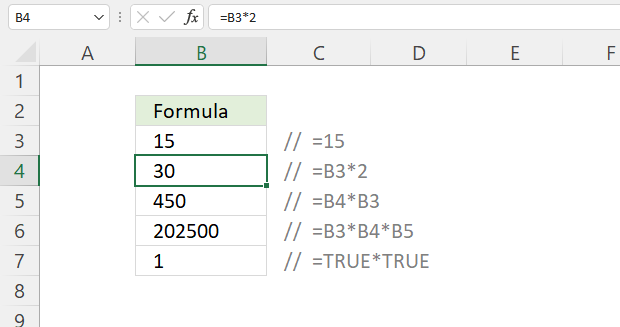This example shows how to multiply a cell value with a constant.

Formula in cell B4:

=B3*2

### Explaining formula

#### Step 1 - Cell reference, asterisk, and the constant

B3*2

Cell B3 is a relative cell reference meaning it will change accordingly if you copy cell B4 and paste to another cell on the worksheet.

Tip! How to toggle between relative and absolute references
Select B3 in the formula with the mouse and then press F9 to convert the relative cell reference to an absolute cell reference. An absolute cell reference has dollar signs before the column letter and row number like this \$B\$3.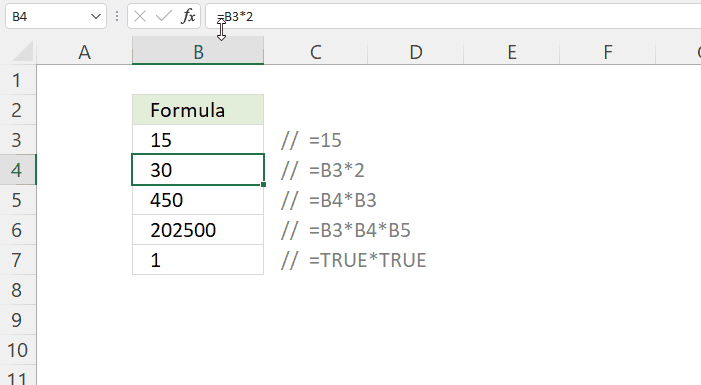An absolute cell reference stays the same when you copy the cell and paste into other cells.

The asterisk character performs multiplication between two numbers in an Excel formula.

2 is a constant.

#### Step 2 - Evaluate the formula

B3*2

becomes

15*2

and returns 30 in cell B4

## 3. How to multiply cell values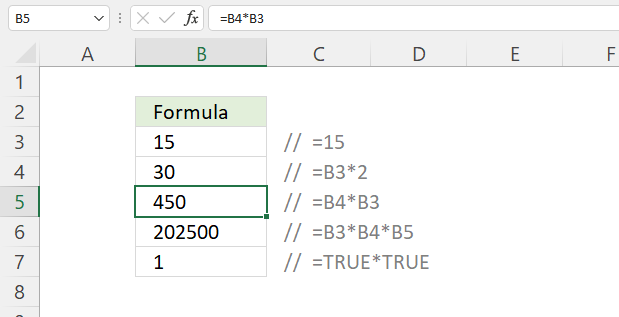The asterisk character lets you multiply two cell values using cell references.

Formula in cell B5:

=B4*B3

B4*B3

becomes

30*15

and returns 450

## 4. How to multiply multiple cell values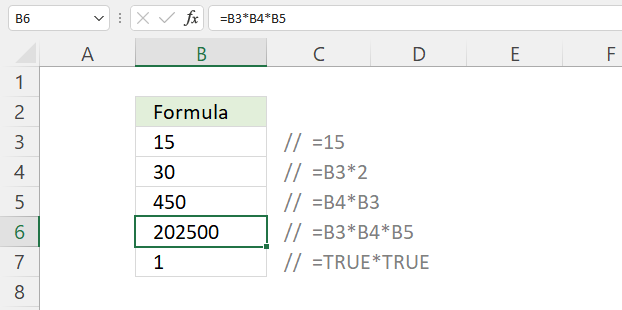You can use the asterisk character almost as many times as you like in an Excel formula. This example shows how to multiply three cell values.

Formula in cell B5:

=B3*B4*B5

### Explaining formula

B3*B4*B5

becomes

15*30*450

and returns 202500

There is an easier way to multiply many cell values that saves you time and energy, Excel has a function for this, read the next section.

## 5. How to multiply values using an Excel function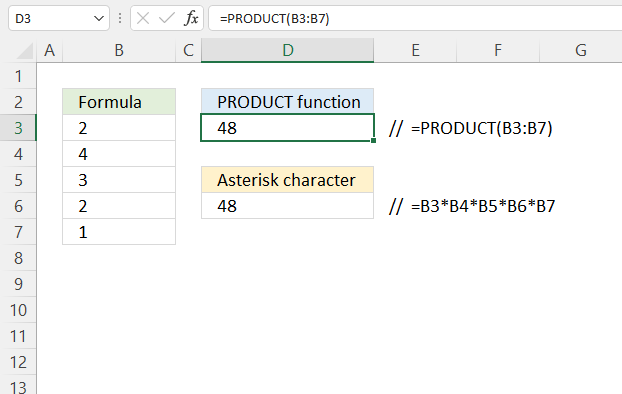The PRODUCT function lets you multiply multiple cell values easily.

Formula in cell D3:

=PRODUCT(B3:B7)

### Explaining formula

#### Step 1 - Populate arguments

The PRODUCT function returns the product of the numbers given in the argument.

Function syntax: PRODUCT(number1, [number2], ... )

number1 - B3:B7

PRODUCT(B3:B7)

#### Step 2 - Evaluate the PRODUCT function

PRODUCT(B3:B7)

becomes

PRODUCT({2;4;3;2;1})

and returns 48

2*4*3*2*1 equals 48

Hold CTRL key while selecting cells with the mouse to quickly add specific cells or cell ranges to the PRODUCT function.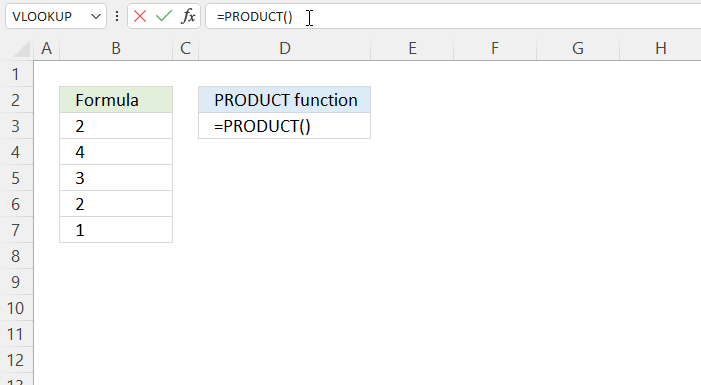## 6. How to multiply functions in an Excel formula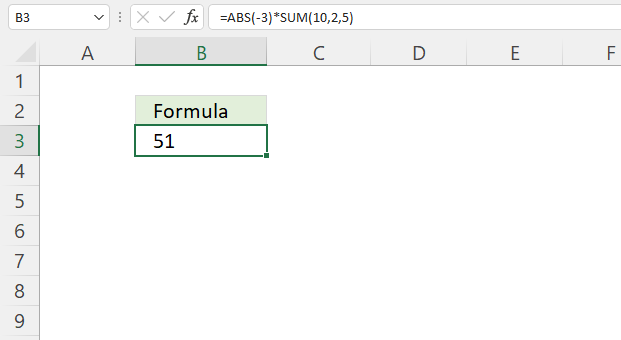This example shows how to multiply two different Excel functions.

Formula in cell D3:

=ABS(-3)*SUM(10,2,5)

### Explaining formula

#### Step 1 - Evaluate ABS function

The ABS function converts negative numbers to positive numbers.

Function syntax: ABS(number)

ABS(-3)

returns 3

#### Step 2 - Evaluate SUM function

The SUM function allows you to add numerical values, the function returns the sum in the cell it is entered in. The SUM function is cleverly designed to ignore text and boolean values, adding only numbers.

Function syntax: SUM(number1, [number2], ...)

SUM(10,2,5)

returns 17

#### Step 3 - Multiply numbers

ABS(-3)*SUM(10,2,5)

becomes

3*17

equals 51

## 7. How to multiply boolean values - apply AND logic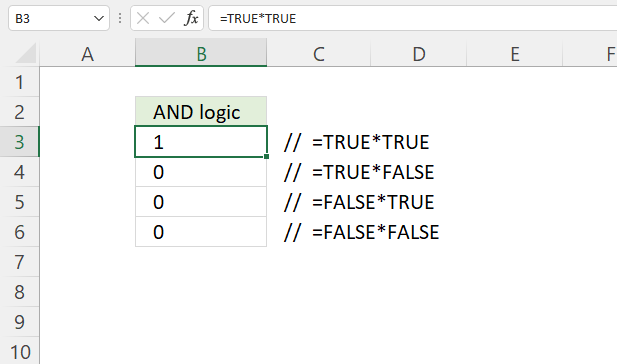The asterisk character lets you multiply both numbers and boolean values in an Excel formula, however, you can't multiply text values.

If you multiply boolean values you are in fact performing AND logic to the calculation and converting the boolean values to their numerical equivalents, here is why.

TRUE * TRUE equals 1 (TRUE)
TRUE * FALSE equals 0 (FALSE)
FALSE * TRUE equals 0 (FALSE)
FALSE * FALSE equals 0 (FALSE)

AND logic meaning both or more boolean values must all be TRUE in order to return TRUE.

## 8. Arithmetics - order of operation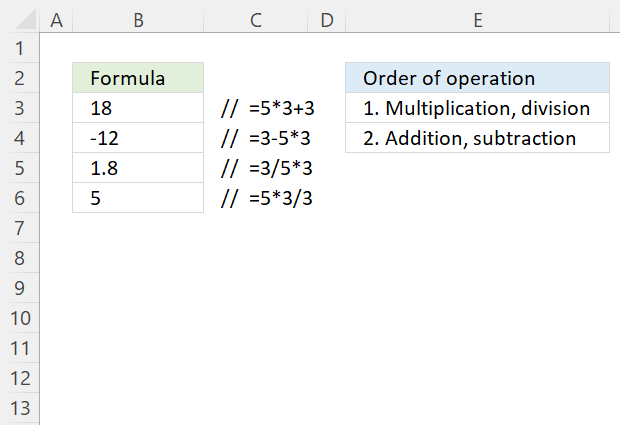This example shows that multiplication and division are calculated before addition and subtraction.

Formula in cell B3:

=5*3+3

5*3

equals 15

15 + 3

equals 18

### 8.1 Multiplication and subtractionFormula in cell B3:

=3-5*3

3-5*3

becomes

3-15

equals -12

## 9. Partial match VLOOKUP - asterisk character (wildcard)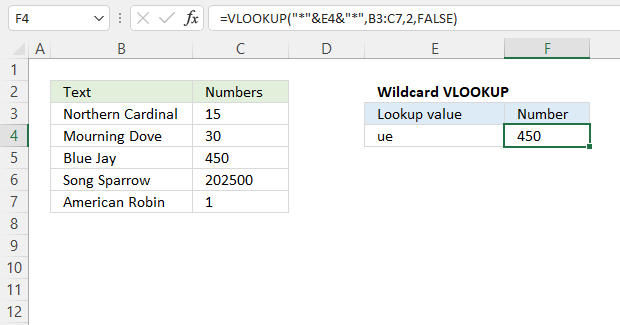The asterisk character lets you also perform a wildcard match, the asterisk matches zero to multiple numbers of characters in Excel. This example demonstrates two asterisks, one before and one after the lookup value.

This makes the VLOOKUP function look for a cell value that contains the search string or in other words a partial match.

Formula in cell F4:

=VLOOKUP("*"&E4&"*",B3:C7,2,FALSE)

This formula looks for the specified value in cell E4 in cell range B3:B7 and returns the adjacent value in cell range C3:C7 on the same row.

Note, the VLOOKUP function returns only the first match from top to bottom in B3:B7 even if there are multiple values matching.

### Explaining formula

#### Step 1 - Append asterisks to cell reference

The ampersand character lets you concatenate values in an Excel formula, this example demonstrates how to append asterisks to a particular cell reference.

"*"&E4&"*"

The double quotes are needed in order to encapsulate text values in an Excel formula.

#### Step 2 - Populate the VLOOKUP arguments

The VLOOKUP function lets you search the leftmost column for a value and return another value on the same row in a column you specify.

Function syntax: VLOOKUP(lookup_value, table_array, col_index_num, [range_lookup])

lookup_value - "*"&E4&"*"
table_array - B3:C7
col_index_num - 2
[range_lookup] - FALSE

becomes

VLOOKUP("*"&E4&"*",B3:C7,2,FALSE)

#### Step 3 - Evaluate the VLOOKUP function

VLOOKUP("*"&E4&"*",B3:C7,2,FALSE)

becomes

VLOOKUP("*ue*", {"Northern Cardinal", 15;"Mourning Dove", 30;"Blue Jay", 450;"Song Sparrow", 202500;"American Robin", 1}, 2, FALSE)

and returns

450